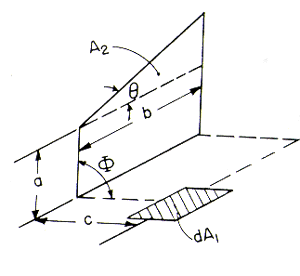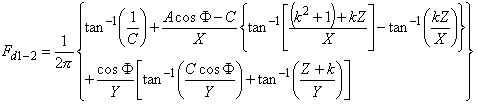>

# SECTION B Factors From Differential Elements to Finite Areas

## B-6: Planar element to rectangle with right triangle added. Vertex of added triangle at end of rectangle nearest element.Definitions: Z=A-C cosΦ; A=a/b; C=c/b; k=tanΘ; X=[(k2+1)C2sin2Φ+Z2]1/2; Y=(1+C2sin2Φ)1/2

Governing equation:To download a copy of this equation in MS Word 97 Format, click HERE.